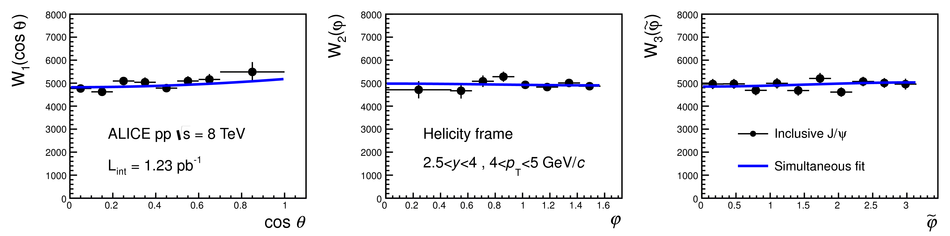Figure 2

 Acceptance corrected angular distributions of J/$\psi$ reconstructed in the di-muon decay channel $W_1(\cos\theta)$, $W_2(\varphi)$ and $W_3(\widetilde{\varphi})$ in the helicity frame for the transverse momentum interval $4 < p_{\rm T} < 5$~\GeVc, together with the results of the simultaneous fit (see text for details). Vertical bars correspond to statistical uncertainties.